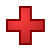Games
Problems
Go Pro!

# Addition by 8

Quizzes > Mathematics > Arithmetic > AdditionPractice your addition facts with this simple math quiz at The Problem Site.

When you click Start Quiz, multiple-choice questions will appear one at a time. Choose the correct answers, and find out how much you know!
Quiz by Mr. TwitchellAssign this quiz
Click here to assign this quiz to your students.

# Understanding Coronavirus Spread

A Question and Answer session with Professor Puzzler about the math behind infection spread.

# Blogs on This SiteReviews and book lists - books we love!The site administrator fields questions from visitors.

# Questions

Here are some of the questions you may encounter in the quiz Addition by 8.

8 + 5 = ?
8 + 7 = ?
8 + 8 = ?
8 + 4 = ?
8 + 2 = ?
8 + 3 = ?
8 + 0 = ?
8 + 6 = ?
8 + 9 = ?
8 + 1 = ?Like us on Facebook to get updates about new resources